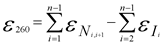w10.0.27 | c9.0.90.05
PROD | u7.5.14

Find the answers to all our products and services by clicking the links below.

How do I calculate the extinction coefficient of an oligonucleotide?

For your convenience, please use our Oligo Analysis Tool or apply the formula below:

The extinction coefficient at 260 nm (e260) is a unique physical property of each oligonucleotide. It is defined as the absorbance at 260 nm of a 1 M aqueous solution measured at 20 °C in an optical cell with 1 cm pathway (Lambert-Beer's law).
Purinic bases show a higher absorption (OD260) than pyrimidinic bases. Interactions between neighbouring bases, as well as modifications that absorb at 260nm, also influence optical absorbance. As a consequence, the extinction coefficient strongly depends on oligonucleotide sequence and composition.

The most accurate method to calculate the extinction coefficient of an oligonucleotide is based on the nearest-neighbor model. The average error of calculated extinction coefficients was shown to be around 4 % using following formular:How do I calculate the molar amount N of an oligo from the OD value?

For your convenience, please use our Oligo Analysis Tool or apply the formula below:

N [nmol] = OD x 100 / 1.54 x nA + 0.75 x nC + 1.17 x nG + 0.92 x nT

n = number of nucleosides of the type X

Example:
Sequence: GAA ATG AGT GCT CAT CAC TAC TTC CGC (27mer)

nA=7; nC=8; nG=5; nT=7
OD=12 (measured)

N [nmol] = 12 x 100 / 1.54 x 7 + 0.75 x 8 + 1.17 x 5 + 0.92 x 7
= 41.3

How do I calculate the mass amount m from the molar amount N of an oligo?

For your convenience, please use our Oligo Analysis Tool or apply the formula below:

M[µg] = N x MW / 1000
MW = Molecular weight

Example:
Sequence: GAA ATG AGT GCT CAT CAC TAC TTC CGC (27mer)

nA=7; nC=8; nG=5; nT=7
MW (calculated) = 8219 g/mol
N= 41.3 nmol

M[µg] = 41.3 x 8219 / 1000 = 339

How do I calculate the molecular weight M of an oligonucleotide?

For your convenience, please use our Oligo Analysis Tool or apply the formula below:

MW [g/mol]= 249.23 x nA + 240.21 x nT + 265.23 x nG + 225.20 x nC + 63.98 x (s-1) + 2.02

n = number of nucleosides of the type X
s = number of all nucleosides per sequence

Example:
Sequence: GAA ATG AGT GCT CAT CAC TAC TTC CGC (27mer)

nA=7; nC=8; nG=5; nT=7

MW [g/mol]= 249.23 x 7 + 240.21 x 7 + 265.23 x 5 + 225.20 x 8+ 63.98 x (s-1) + 2.02 = 8219.33

How do I calculate the melting temperature TM of an oligo?

The melting temperature TM, characterises the stability of the DNA hybrid formed between an oligonucleotide and its complementary strand.

For your convenience, please use our Oligo Analysis Tool or apply the formula below:

Sequences with 15 or less bases
TM [°C] = 2(nA + nT) + 4(nG + nC)

Sequences with more than 15 bases
TM [°C] = 69.3 + [41(nG + nC) / s - (650 / s)]

n = number of nucleosides of type X
s = number of all nucleosides per sequence

Example:
Sequence: GAA ATG AGT GCT CAT CAC TAC TTC CGC (27mer)

nA=7; nC=8; nG=5; nT=7 s= 27

Sequences with 15 or less bases
TM [°C] = 2(7 + 7) + 4(5 + 8) = 80.0

Sequences with more than 15 bases
TM [°C] = 69.3 + [41(5 + 8) / 27] - (650 / 27) = 69.3 + 19.7 - 24.0 = 65.0

How do I calculate the annealing temperature TA?

PCR                  TA [°C] = [(TM1 + TM2) / 2] - 3

Sequencing        TA [°C] = TM + 3